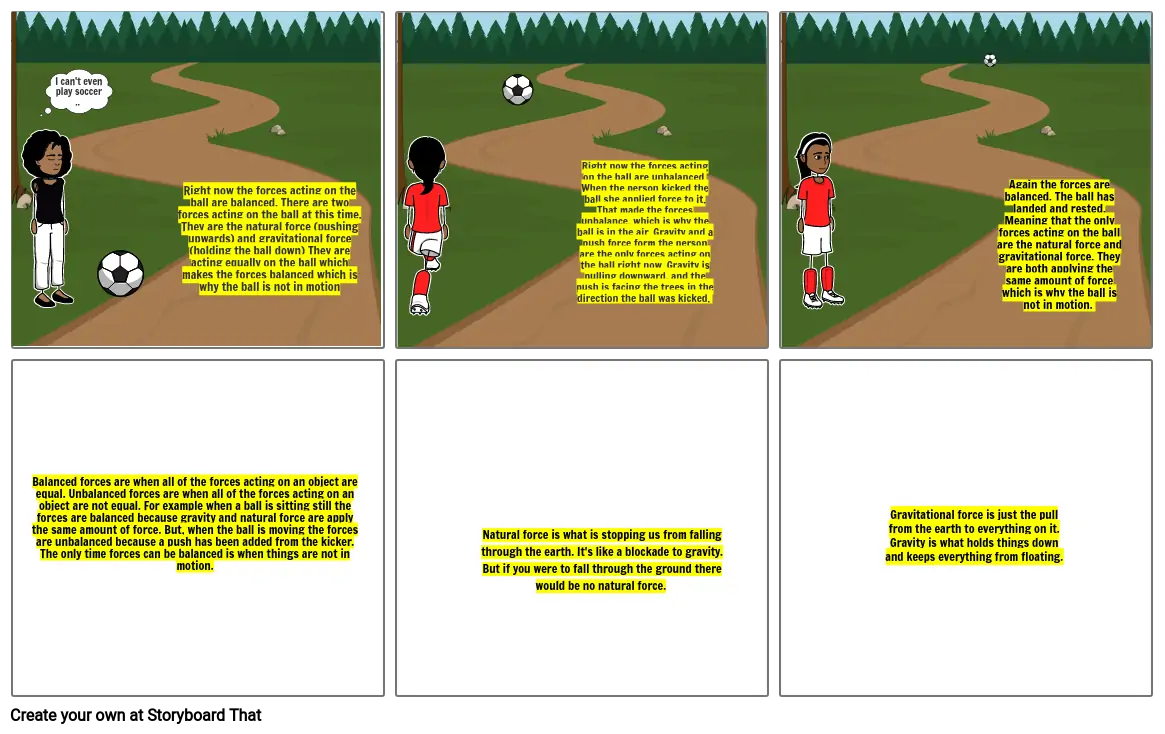# Physics#### Storyboard Text

• I can't even play soccer ..
• ﻿Right now the forces acting on the ball are balanced. There are two forces acting on the ball at this time. They are the natural force (pushing upwards) and gravitational force (holding the ball down) They are acting equally on the ball which makes the forces balanced which is why the ball is not in motion
• ﻿﻿Right now the forces acting on the ball are unbalanced. When the person kicked the ball she applied force to it. That made the forces unbalance, which is why the ball is in the air. Gravity and a push force form the person are the only forces acting on the ball right now. Gravity is pulling downward, and the push is facing the trees in the direction the ball was kicked.
• ﻿Again the forces are balanced. The ball has landed and rested. Meaning that the only forces acting on the ball are the natural force and gravitational force. They are both applying the same amount of force which is why the ball is not in motion.
• Balanced forces are when all of the forces acting on an object are equal. Unbalanced forces are when all of the forces acting on an object are not equal. For example when a ball is sitting still the forces are balanced because gravity and natural force are apply the same amount of force. But, when the ball is moving the forces are unbalanced because a push has been added from the kicker. The only time forces can be balanced is when things are not in motion.
• Natural force is what is stopping us from falling through the earth. It's like a blockade to gravity. But if you were to fall through the ground there would be no natural force.
• ﻿Gravitational force is just the pull from the earth to everything on it. Gravity is what holds things down and keeps everything from floating.
##### Over 20 Million Storyboards Created